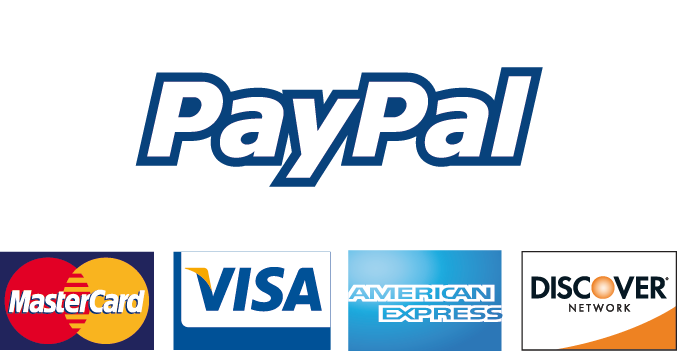We're Open
+44 7340 9595 39
+44 20 3239 6980

# Which project(s) should you accept if the discount rate is 7 percent?## Information

• Post Date 2018-11-05T12:16:08+00:00
• Post Category Essays

No Plagiarism Guarantee - 100% Custom Written

# Cash Flow Writing Assignments: Projects With Positive NPV

INSTRUCTIONS:

Writing assignments (Due Saturday as a Word or Excel file) Show ALL Work You are considering two mutually exclusive projects with the following cash flows. Which project(s) should you accept if the discount rate is 7 percent? What if the discount rate is 10 percent?

Radiology Associates is considering an investment which will cost \$259,000. The investment produces no cash flows for the first year. In the second year, the cash inflow is \$58,000. This inflow will increase to \$150,000 and then \$200,000 for the following two years before ceasing permanently. The firm requires a 14 percent rate of return and has a required discounted payback period of three years. Accept or reject this project? Why?

CONTENT:

Name

Institution

Date

Writing assignments (Due Saturday as a Word or Excel file) Show ALL Work You are considering two mutually exclusive projects with the following cash flows. Which project(s) should you accept if the discount rate is 7 percent? What if the discount rate is 10 percent?

At 7 % the NPV for project A is cash inflows – cash outflows, (\$ 360,000* 0.8163) - \$275,0000= \$18,868

Project B, NPV= \$113,600*0.0.9346+ (\$81,900*0.8734) + (\$47,000*0.8163) - \$202,000= \$14,068

At 10 % NPV project A= (\$360,000*0.7513) - \$ 360,000= (\$4,532)

NPV Project B = (\$116,600*0.9091) + (\$81,900*0.8264) + (\$47,000*0.7513) - \$202,000= \$4,267

* At 7% choose Project A with higher NPV

* At 10% choose project B with higher NPV

1 Radiology Associates is considering an investment which will cost \$259,000. The

...

## Price: £ 99

100% Plagiarism Free & Custom Written, Tailored to your instructions